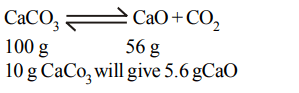## Some Basic Concepts of Chemistry Questions and Answers Part-3

1. The simplest formula of a compound containing 50% of element X (atomic mass 10) and 50% of element Y (atomic mass 20) is:
a) XY
b) $XY_{3}$
c) $X_{2}Y$
d) $X_{2}Y_{3}$

Explanation: 50% x atomic mass 10, 50% y atomic mass 20. Relative number of atoms of X = $\frac{50}{10}$ = 5 and that Y = $\frac{50}{20}$ = 2.5
Simple Ratio 2 : 1. Formula X2Y

2. The hydrogen phosphate of certain metal has formula  $MHPO_{4}$  . The formula of metal chloride would be
a) MCl
b) $M_{2}CI_{2}$
c) $MCl_{2}$
d) $MCl_{3}$

Explanation: Formula of metal phosphate is M++H+PO4 --- . Valency of metal + 2. Hence metal chloride is MCl2

3. A compound contains atoms of three elements as A, B and C. If the oxidation number of A is +2, B is +5 and that of C is –2, the possible formula of the compound is
a) $A_{3}\left(B_{4}C\right)_{2}$
b) $A_{3}\left(BC_{4}\right)_{2}$
c) $ABC_{2}$
d) $A_{2}\left(BC_{3}\right)_{2}$

Explanation: Sum of oxidation states must be equal to zero which is given by A3(BC4)2 = A3B2C8 (+ 6 + 10 – 16 = 0)

4. If 0.44 g of a colourless oxide of nitrogen occupies 224 ml at 1520 mm Hg and 273°C, then the compound is
a) $NO_{2}$
b) $N_{2}O$
c) $NO_{4}$
d) $N_{2}O_{2}$

Explanation:5. The mass of a molecule of water is
a) $3 × 10^{-25}kg$
b) $3 × 10^{-26}kg$
c) $1.5 × 10^{-26}kg$
d) $2.5 × 10^{-26}kg$

Explanation:6. Number of moles of $KMnO_{4}$   required to oxidize one mole of $Fe\left(C_{2}O_{4}\right)$   in acidic medium is
a) 0.167
b) 0.6
c) 0.2
d) 0.4

Explanation:7. $100cm^{3}$ of 0.1 N HCl is mixed with $100cm^{3}$  of 0.2 N NaOH solution. The resulting solution is
a) 0.1 N and the solution is basic
b) 0.1 N and the solution is acidic
c) 0.05 N and the solution is basic
d) 0.05 N and the solution is acidic

Explanation:8. For preparing 0.1 N solution of a compound from its impure sample of which the percentage purity is known, the weight of the substance required will be
a) less than the theoretical weight
b) more than the theoretical weight
c) same as the theoretical weight
d) none of these

Explanation: More than theoretical weight since impurity will not contribute

9. $10 gCaCO_{3}$   gives on strong heating $CO_{2}$. It gives quicklime (in grams)
a) 5g
b) 4.4 g
c) 5.6 g
d) 4 g